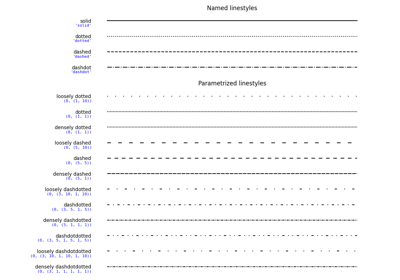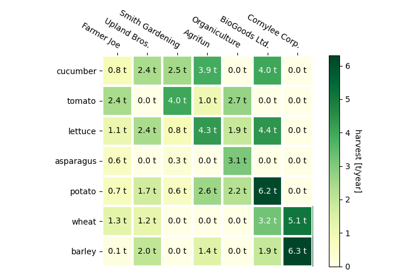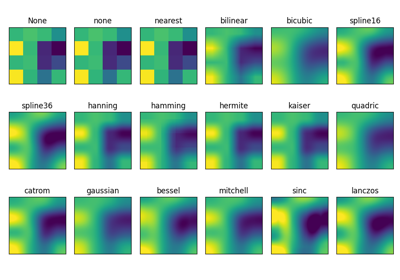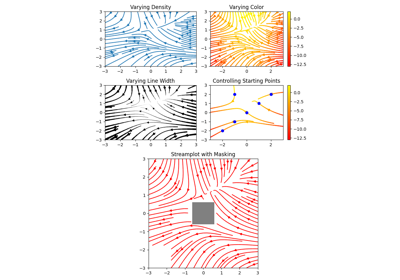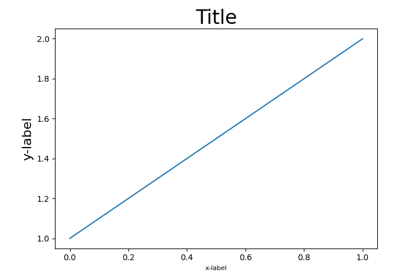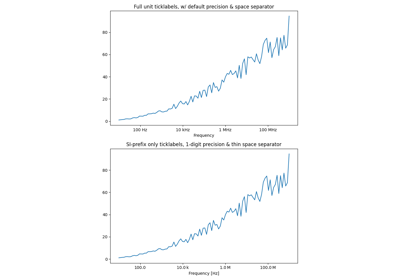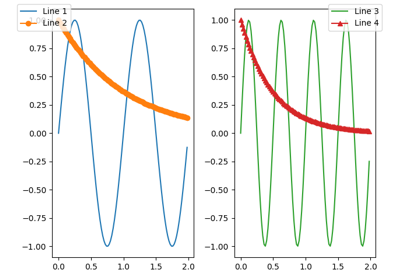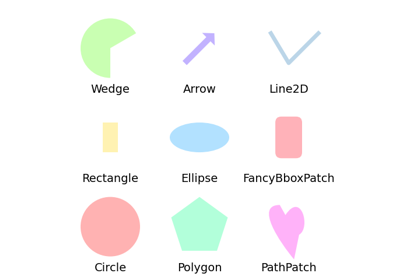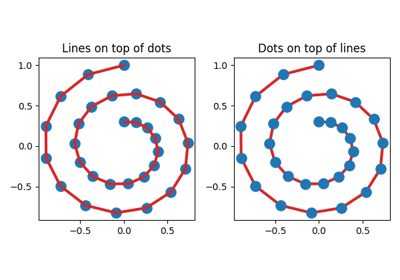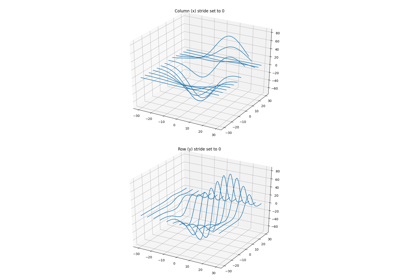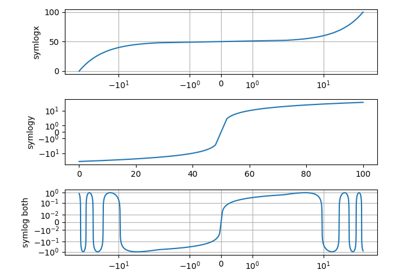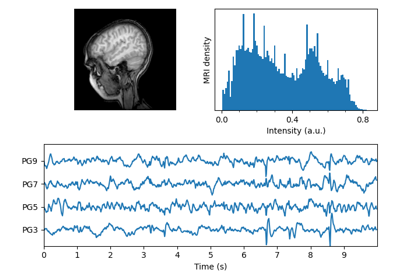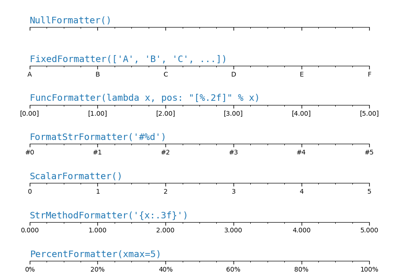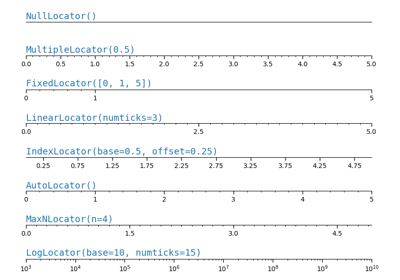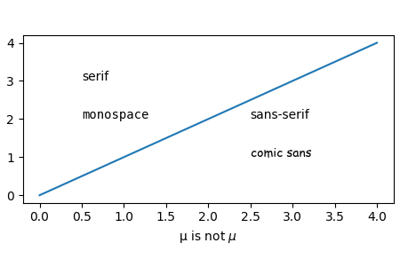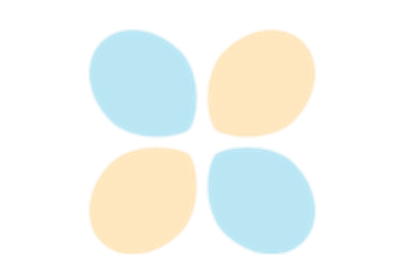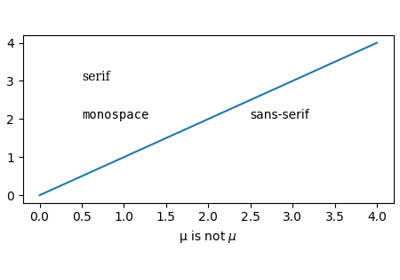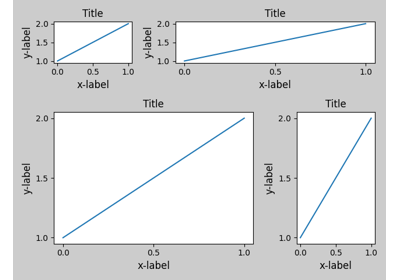# matplotlib.pyplot.tight_layout¶

matplotlib.pyplot.tight_layout(pad=1.08, h_pad=None, w_pad=None, rect=None)[source]

Parameters: padfloatPadding between the figure edge and the edges of subplots, as a fraction of the font size. h_pad, w_padfloat, optionalPadding (height/width) between edges of adjacent subplots, as a fraction of the font size. Defaults to pad. recttuple (left, bottom, right, top), optionalA rectangle (left, bottom, right, top) in the normalized figure coordinate that the whole subplots area (including labels) will fit into. Default is (0, 0, 1, 1).

## Examples using matplotlib.pyplot.tight_layout¶